SSC BOARD PAPERS IMPORTANT TOPICS COVERED FOR BOARD EXAM 2024

### Lenses Class 10th Science And Technology Part 1 MHB Solution

##### Class 10th Science And Technology Part 1 MHB Solution

###### Exercise
Question 1.

Match the columns in the following table and explain them.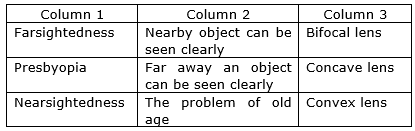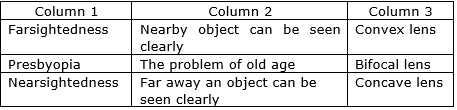(i) Farsightedness

● It is also known as Hypermetropia.

● In this defect of vision, the closer objects are blurred

● It occurs due to the flat cornea or due to the incorrect curvature of the eye lens.

(ii) Nearsightedness

● It is also known as Myopia

● In this defect vision of distant objects are blurred.

● It occurs due to due to defect in eye lense as the image is formed in front of the retina and not on it.

● It is most common types of an eye defect.

Question 2.

Draw a figure explaining various terms related to a lens.

i. OpticalCentre:

The center point of a lens that lies on the principal axis of the lens is called its optical center. The optical center is represented by letter C.

ii. Principal Axis

The principal axis is a straight line passing through the optical center and the center of curvature of two surfaces of a lens. It is represented by letter P

iii. Principal Focus

The principal focus is a point on its principal axis to which the light rays parallel to the principal axis converge (in case of convex lens) or appear to diverge (in case of the concave lens) after passing through it. It is represented by letter F

iv. Focal Length

The focal length of a lens is the distance between its optical center and principal focus. It is represented by letter f

F = Principal focus

2F= Pole of the lens

C = Optical centre

P = Principal axis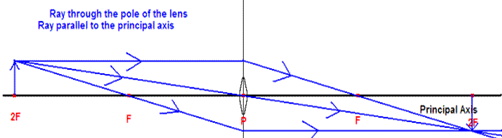Question 3.

At which position will you keep an object in front of a convex lens so as to get a real image of the same size as the object? Draw a figure.

In case of convex lens when the object is at “2F1”(twice of focal length), a real, inverted image of the same size is formed at “2F2

Explanation:

When the object is placed at the center of curvature of a lens then a ray of light AO which is parallel to the principal axis after refraction pass through the focus F along the direction OF. While the other ray AC pass through the optical center C and goes straight without any deviation. These two refracted light rays intersect each other at point A’, on the other side of the lens at the center of curvature 2F. so, the image A’B’ formed in this case is at the center of curvature, of the same size as the object, real and inverted.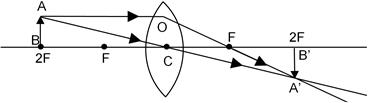Question 4.

Give scientific reasons:

a. Simple microscope is used for watch repairs.

b. One can sense colours only in bright light.

c. We cannot clearly see an object kept at a distance less than 25 cm from the eye.

(a) A simple microscope used in repairing watches has a convex lens of small focal length with magnification capacity up to 20 times. Therefore, it is used by watch repairers to check the smallest parts of the watch clearly and it does not cause any strain on eyes.

(b) We can sense colours only in light because when it gets dark the cones ability to respond to light is lost and the rods continue to respond to available light. However cones cannot see color, everything looks like shades of black and white and gray.

(c) Eyes can see its distant and close objects. It adjusts its focal length by contracting or relaxing ciliary muscles to see the objects. The ciliary muscles cannot be contracted below a certain minimum limit. Therefore we cannot see an object kept at a distance less than 25 cms from the eye.

Question 5.

Explain the working of an astronomical telescope using refraction of light.

In the astronomical telescopes the image is formed by bending of light also known as refraction so these telescopes are known as refracting telescopes. It is used to see the magnified images of heavenly bodies like stars, planets, etc.

The construction of the telescope is given as:

An astronomical telescope is made up of two convex lenses :

i) an objective lens O and,

ii) an eye piece E.

The focal length fo of the objective lens of astronomical telescope is large as compared to the focal length fe of the eye piece.

Working of the telescope:

The ray diagram to show the working of the astronomical telescope is shown in figure below: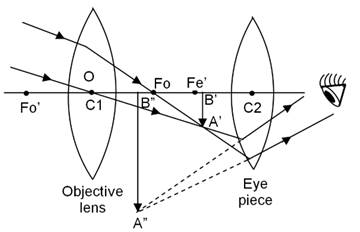i. A parallel beam of light from a star or a satellite falls on the objective lens of the telescope.

ii. The objective lens forms a real, inverted and diminished image A’B’ of the heavenly body.

iii. This image (A’B’) now acts as an object for the eye piece E, whose position is adjusted so that the image lies between the focus fe’ and the optical centre C2 of the eye piece.

iv. Now the eye piece forms a virtual, inverted and highly magnified image of object at infinity. When the final image of an object is formed at infinity, the telescope is said to be in ‘normal adjustment’.

It should be noted that, the final image of object (such as stars, planets or satellites) formed by an astronomical telescope is always inverted with respect to the object. But it does not matter whether the image formed by an astronomical telescope is inverted or not, as all the heavenly bodies are usually spherical is shape. Astronomical telescopes bend light called as refraction. Due to this refraction the light rays which are parallel, converge at a focal point and those lines that are not parallel converge upon focal plane. The telescope converts a bundle of parallel rays and makes angle α and angle β with a second par

allel bundle. The ratio of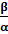gives angular magnification to the telescope.

Question 6.

Distinguish between:

Farsightedness and Nearsightedness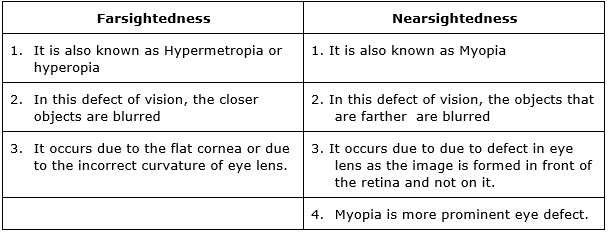The diagram is given below: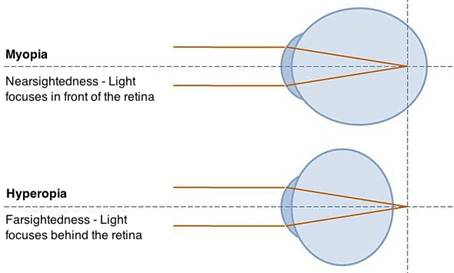Question 7.

Distinguish between:

Concave lens and Convex Lens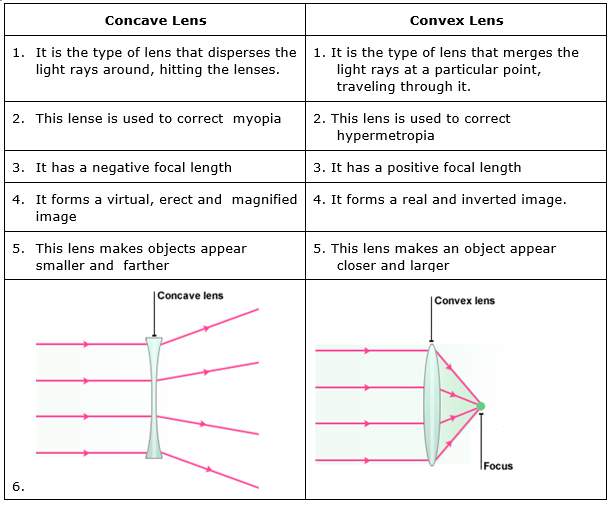Question 8.

What are the function of iris and the muscles connected to the lens in the human eye?

The function of iris - Iris is thin circular structure in the eye. It controls the diameter and size of the pupil and affects the amount of light entering the eye.

Functionof ciliary muscles:

Ciliary muscles surround the lens and hold the lens in place. They also play an important role in vision. They control accommodation for viewing objects at varying distances and regulates the flow of aqueous humour into Schlemm's canal.

Question 9.

Solve the following examples.

The doctor has prescribed a lens having power +1.5 D. What will be the focal length of the lens? What is the type of the lens and what must be the defect of vision?

Power of lens is defined as the reciprocal of its focal length so

1.5 = 1/f

1.5f = 1

⇒ f = 1/1.5

⇒ f= 0.67m

As the focal length is positive so the lens prescribed is positive and has positive power. It is a convex lens. The defect of the vision is Hypermetropia.

Question 10.

Solve the following examples.

5 cm high object is placed at a distance of 25 cm from a converging lens of focal length of 10 cm. Determine the position, size and type of the image.

As per the given information

Height of the object is 5 cms

Focal length f is 10 cms

Object distance u = 25 cms

Image distance v= ?

Height of the image h = ?

⇒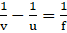⇒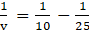⇒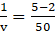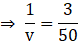V = 50/3 =16.66 cms

The image formed will be real and inverted on the other side of the lens at a distance of 16.66 cms from its optical centre.

Now

m= v/u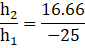Where h2 is the height of the object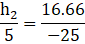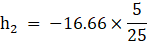⇒ h2 = 3.33cms

Therefore from above we can conclude an inverted image of 3.33cm will be formed.

Question 11.

Solve the following examples.

Three lenses having power 2, 2.5 and 1.7 D are kept touching in a row. What is the total power of the lens combination?

As per the given question three lenses having power 2, 2.5 and 1.7 D are kept touching in a row.

So resultant power will be

P=P1+P2+P3

P= 2+2.5+1.7

P= 6.2D

The resultant combination 6.2D is of convex lens and it will produce a converging effect.

Lens Formula, Magnification and Sign Convention

Question 12.

Solve the following examples.

An object kept 60 cm from a lens gives a virtual image 20 cm in front of the lens. What is the focal length of the lens? Is it a converging lens or diverging lens?

As per the question

The object distance is u=-60cm

Virtual image is v =-20cm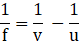⇒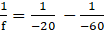⇒ f= -30cm

From above we can conclude that as the focal length is negative it is diverging in nature and is a concave lens.